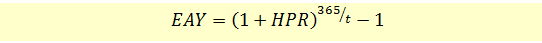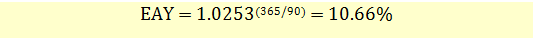# Effective Annual Yield for Money Market Instruments

For a money market instrument such as a T-bill, Effective Annual Yield is the annualized value of the Holding Period Return and is calculated using the following formula:In our T-bill example, the HPR was 2.53%. If the holding period was 90 days, we can calculate the effective annual yield as follows: